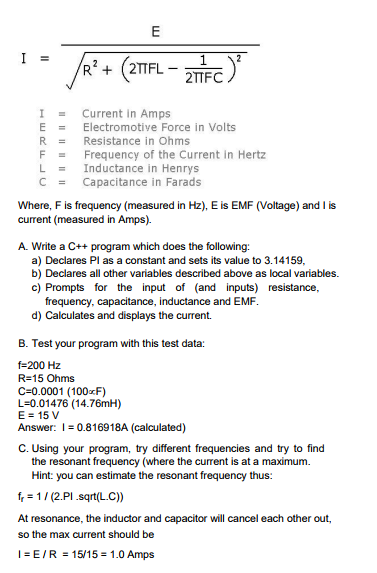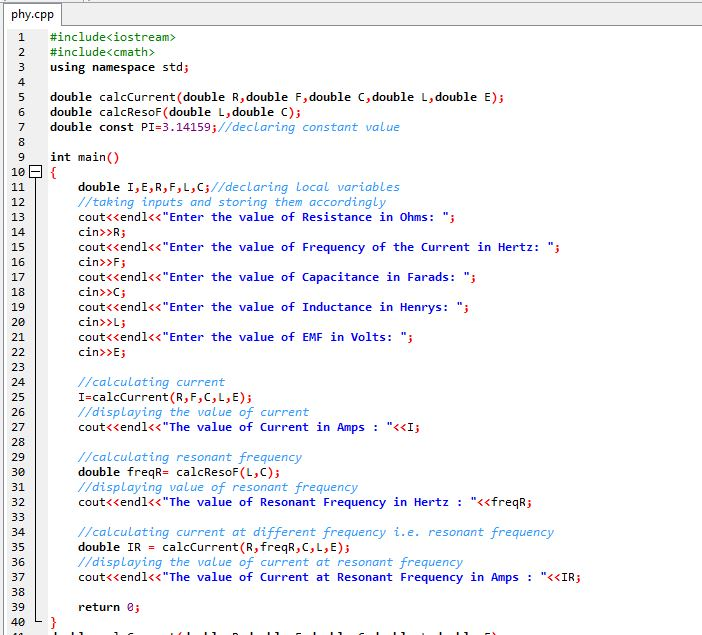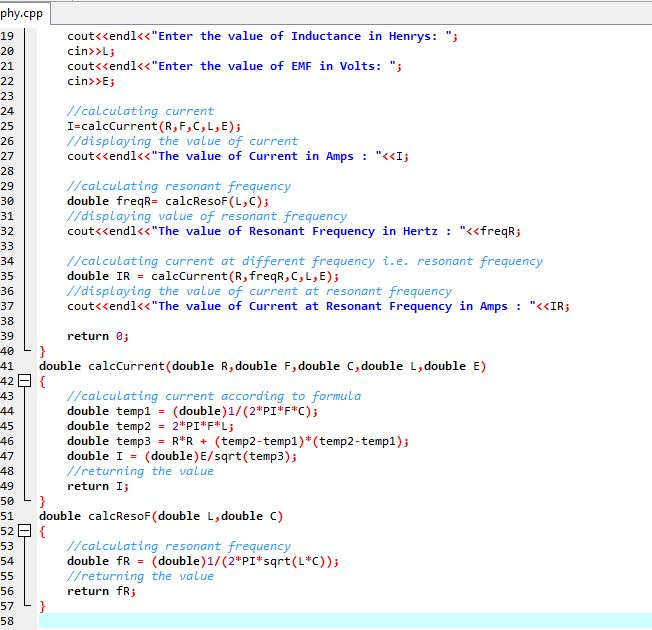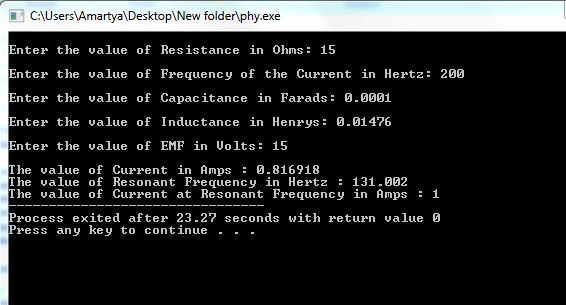# Question & Answer: I = current in Amps E = Electromotive Force in Volts R = Resistance in Ohms F = Frequency of the Current…..I = E/squareroot R^2 + (2pi FL – 1/2pi FC)^2 I = current in Amps E = Electromotive Force in Volts R = Resistance in Ohms F = Frequency of the Current in Hertz L = Inductance in Henrys C = Capacitance in Farads Where, F is frequency (measured in Hz), E is EMF (Voltage) and I is current (measured in Amps). A. Write a C++ program which does the following: a) Declares Pl as a constant and sets its value to 3.14159, b) Declares all other variables described above as local variables. c) Prompts for the input of (and inputs) resistance frequency, capacitance, inductance and EMF. d) Calculates and displays the current. B. Test your program with this test data: f = 200 Hz R = 15 Ohms C = 0.0001 (100 infinity F) L = 0.01476 (14.76mH) E = 15 V Answer: I = 0.816918A (calculated) C. Using your program, try different frequencies and try to find the resonant frequency (where the current is at a maximum. F_r = 1/(2.PI. squareroot (L.C)) At resonance, the inductor and capacitor will cancel each other out, so the max current should be I = E/R = 15/15 = 1.0 Amps

Don't use plagiarized sources. Get Your Custom Essay on
Question & Answer: I = current in Amps E = Electromotive Force in Volts R = Resistance in Ohms F = Frequency of the Current…..
GET AN ESSAY WRITTEN FOR YOU FROM AS LOW AS \$13/PAGE

/*The source code of the above said program is given below in C++ language:*/

#include<iostream>

#include<cmath>

using namespace std;

double calcCurrent(double R,double F,double C,double L,double E);

double calcResoF(double L,double C);

double const PI=3.14159;//declaring constant value

int main()

{

double I,E,R,F,L,C;//declaring local variables

//taking inputs and storing them accordingly

cout<<endl<<“Enter the value of Resistance in Ohms: “;

cin>>R;

cout<<endl<<“Enter the value of Frequency of the Current in Hertz: “;

cin>>F;

cout<<endl<<“Enter the value of Capacitance in Farads: “;

cin>>C;

cout<<endl<<“Enter the value of Inductance in Henrys: “;

cin>>L;

cout<<endl<<“Enter the value of EMF in Volts: “;

cin>>E;

//calculating current

I=calcCurrent(R,F,C,L,E);

//displaying the value of current

cout<<endl<<“The value of Current in Amps : “<<I;

//calculating resonant frequency

double freqR= calcResoF(L,C);

//displaying value of resonant frequency

cout<<endl<<“The value of Resonant Frequency in Hertz : “<<freqR;

//calculating current at different frequency i.e. resonant frequency

double IR = calcCurrent(R,freqR,C,L,E);

//displaying the value of current at resonant frequency

cout<<endl<<“The value of Current at Resonant Frequency in Amps : “<<IR;

return 0;

}

double calcCurrent(double R,double F,double C,double L,double E)

{

//calculating current according to formula

double temp1 = (double)1/(2*PI*F*C);

double temp2 = 2*PI*F*L;

double temp3 = R*R + (temp2-temp1)*(temp2-temp1);

double I = (double)E/sqrt(temp3);

//returning the value

return I;

}

double calcResoF(double L,double C)

{

//calculating resonant frequency

double fR = (double)1/(2*PI*sqrt(L*C));

//returning the value

return fR;

}

/*For better understanding code image and output screenshot is attached here*/